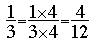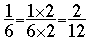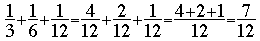SEARCH HOMEMath Central Quandaries & QueriesQuestion from Lisa: As an educator in Adult Education, I am preparing a young man for a US Government test. The equation I have trouble with is explaining the following equation. It asks: If it takes A 3 days to dig a certain ditch, whereas b can dig it in 6 days, and C in 12, how long would it take all three to do the job? I know the equation is 1/3 + 1/6 + 1/12 and it equals = 7/12 but I don't understand how it equals 7/12. This simple math eludes me. So the question is not the final answer of 1 and 5/7 of days but where one comes up with the 7/12? Thank You and Happy New!We have two responses for you

Hi Lisa,

To add fractions we need their denominators to be equal. Thus for your problem you need to find equivalent fractions for 1/3, 1/6 and 1/12 all of which have the same denominator. With these three fractions you can use the denominator 12 sinceandThusPenny

In 12 days, A digs 4 ditches, B digs 2, and C digs 1, so all together they dig 7 ditches. How long does it take them to dig 1 ditch?

ClaudeMath Central is supported by the University of Regina and The Pacific Institute for the Mathematical Sciences.